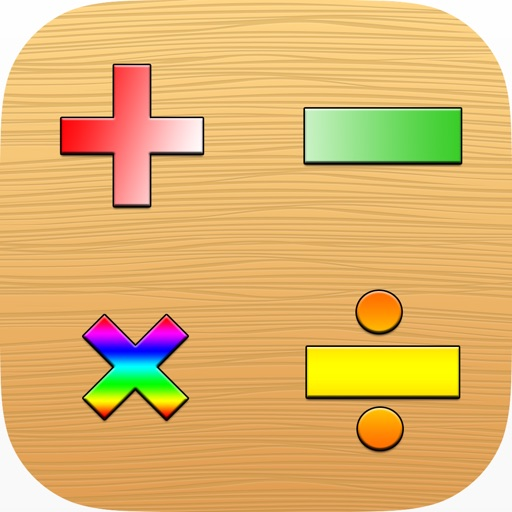## MathPlus: addition, subtraction, multiplication, and division games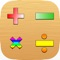# MathPlus : Quotients Math Game Addition, Subtraction, Multiplication, and Division

by Nukorn Samphassri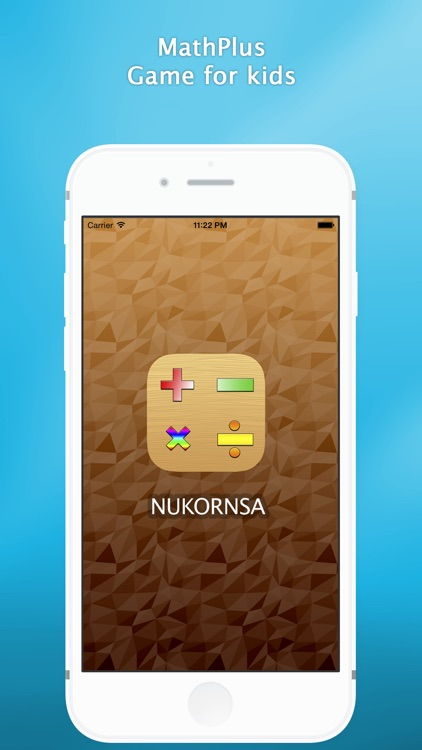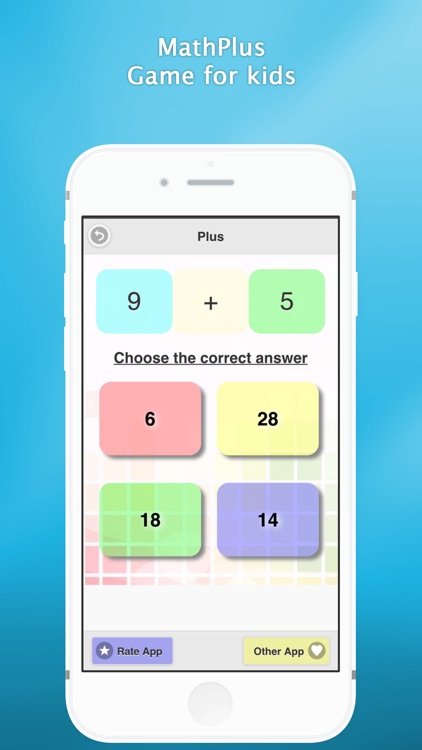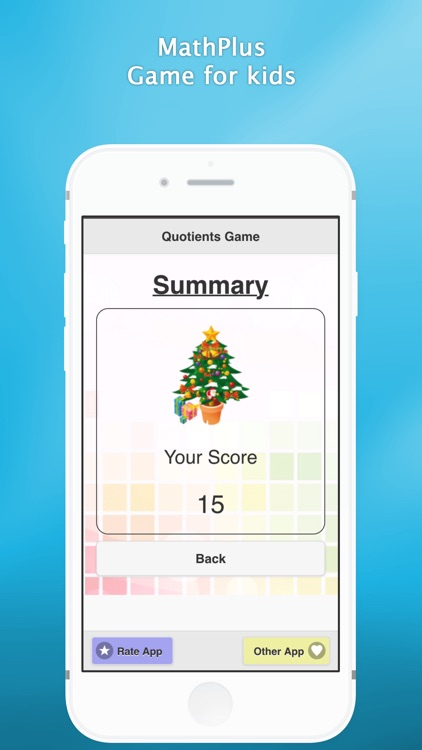MathPlus: addition, subtraction, multiplication, and division games.### App Details

Version
1.1.0
Rating
(5)
Size
17Mb
Genre
Puzzle Education
Last updated
February 23, 2015
Release date
October 7, 2014

### App Store Description

MathPlus: addition, subtraction, multiplication, and division games.
To play games math calculator with addition, subtraction, multiplication, division, simple to play.
For training children to a calculator. And practice with the The answer is in the selection of the selected sound English. Help them learn the English language along well.

The format of the game will be divided into five categories.
1 Addition : plus (+) indicates a positive proposition number only.
2 Subtraction : minus (-) indicates a negative number is given.
3 Multiplication : (x) the multiplication problem only.
4 Division (/) indicates the division problem only.
5 Quotients show Proposition switch more of addition, subtraction, multiplication and division.

Hopefully this will help children develop it even further.

Meet with other application developers from the link below.

http://nukornsa.blogspot.com